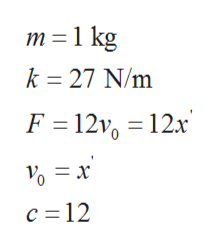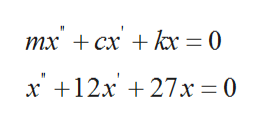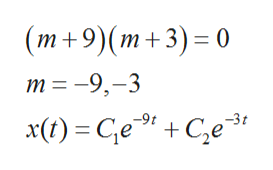A 1-kilogram mass is attached to a spring whose constant is 27 N/m, and the entire system is then submerged in a liquid that imparts a damping force numerically equal to 12 times the instantaneous velocity. Determine the equations of motion if the following is true.(a) the mass is initially released from rest from a point 1 meter below the equilibrium positionx(t)=_________________________________________m(b) the mass is initially released from a point 1 meter below the equilibrium position with an upward velocity of 11 m/sx(t)=___________________________________________m

Question

A 1-kilogram mass is attached to a spring whose constant is 27 N/m, and the entire system is then submerged in a liquid that imparts a damping force numerically equal to 12 times the instantaneous velocity. Determine the equations of motion if the following is true.

(a) the mass is initially released from rest from a point 1 meter below the equilibrium position

x(t)=_________________________________________m

(b) the mass is initially released from a point 1 meter below the equilibrium position with an upward velocity of 11 m/s

x(t)=___________________________________________m

Step 1

Given,help_outlineImage Transcriptionclosem 1 kg k 27 N/m F 12 12x c 12 fullscreen
Step 2

As we know equation used for dampinghelp_outlineImage Transcriptioncloseтх + сх + h 3 0 х +12х + 27х %3D0 fullscreen
Step 3

To solve the give...help_outlineImage Transcriptionclose(m+9)(m+3) 0 m -9,-3 x() - Се " +C,е -9t -3г fullscreen

Want to see the full answer?

See Solution

Want to see this answer and more?

Our solutions are written by experts, many with advanced degrees, and available 24/7

See Solution
Tagged in

Physics